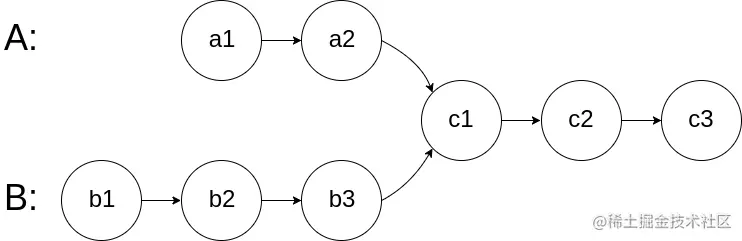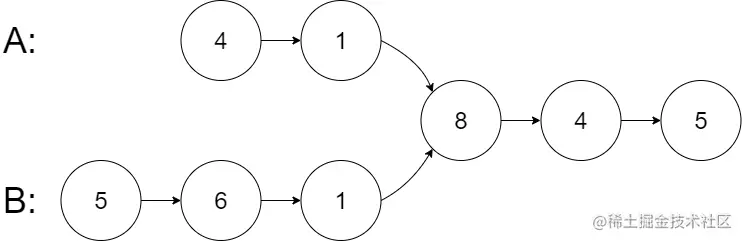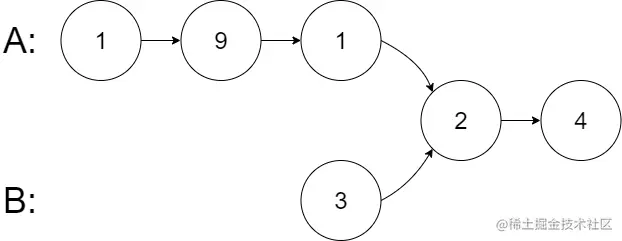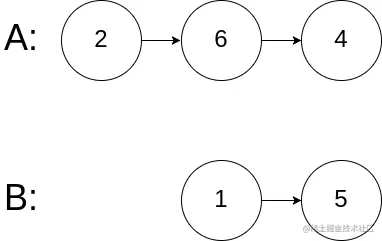# leetcode 160. Intersection of Two Linked Lists （python）

2022-01-30 19:47:56

「 This is my participation 11 The fourth of the yuegengwen challenge 1 God , Check out the activity details ：2021 One last more challenge

### describe

Given the heads of two singly linked-lists headA and headB, return the node at which the two lists intersect. If the two linked lists have no intersection at all, return null.

For example, the following two linked lists begin to intersect at node c1:The test cases are generated such that there are no cycles anywhere in the entire linked structure.

Note that the linked lists must retain their original structure after the function returns.

Custom Judge:

The inputs to the judge are given as follows (your program is not given these inputs):

• intersectVal - The value of the node where the intersection occurs. This is 0 if there is no intersected node.
• listA - The first linked list.
• listB - The second linked list.
• skipA - The number of nodes to skip ahead in listA (starting from the head) to get to the intersected node.
• skipB - The number of nodes to skip ahead in listB (starting from the head) to get to the intersected node.

The judge will then create the linked structure based on these inputs and pass the two heads, headA and headB to your program. If you correctly return the intersected node, then your solution will be accepted.

Follow up: Could you write a solution that runs in O(n) time and use only O(1) memory?

Example 1:``````Input: intersectVal = 8, listA = [4,1,8,4,5], listB = [5,6,1,8,4,5], skipA = 2, skipB = 3
Output: Intersected at '8'
Explanation: The intersected node's value is 8 (note that this must not be 0 if the two lists intersect).
From the head of A, it reads as [4,1,8,4,5]. From the head of B, it reads as [5,6,1,8,4,5]. There are 2 nodes before the intersected node in A; There are 3 nodes before the intersected node in B.
Copy code ``````

Example 2:``````Input: intersectVal = 2, listA = [1,9,1,2,4], listB = [3,2,4], skipA = 3, skipB = 1
Output: Intersected at '2'
Explanation: The intersected node's value is 2 (note that this must not be 0 if the two lists intersect).
From the head of A, it reads as [1,9,1,2,4]. From the head of B, it reads as [3,2,4]. There are 3 nodes before the intersected node in A; There are 1 node before the intersected node in B.
Copy code ``````

Example 3:``````Input: intersectVal = 0, listA = [2,6,4], listB = [1,5], skipA = 3, skipB = 2
Output: No intersection
Explanation: From the head of A, it reads as [2,6,4]. From the head of B, it reads as [1,5]. Since the two lists do not intersect, intersectVal must be 0, while skipA and skipB can be arbitrary values.
Explanation: The two lists do not intersect, so return null.
Copy code ``````

Note:

``````The number of nodes of listA is in the m.
The number of nodes of listB is in the n.
0 <= m, n <= 3 * 10^4
1 <= Node.val <= 10^5
0 <= skipA <= m
0 <= skipB <= n
intersectVal is 0 if listA and listB do not intersect.
intersectVal == listA[skipA] == listB[skipB] if listA and listB intersect.

Copy code ``````

### analysis

According to the meaning , Is to give the head nodes of two linked lists headA and headB , If the two linked lists intersect, let's find the intersecting node , If it doesn't intersect, return directly None Just go . The problem also makes it more difficult for us , We are required to be in O(1) Complete the problem requirements under the level of memory .

The idea is simple , The simplest way is to traverse two linked lists headA and headB Calculate their length difference d , Let the longer one go forward first d Nodes , Then the two linked lists go back at the same time , If there are intersecting nodes, the pointers must be equal , No intersection is found after traversal .

``````class ListNode(object):
def __init__(self, x):
self.val = x
self.next = None

class Solution(object):
"""
:rtype: ListNode
"""
diff = abs(len_a-len_b)
for i in range(diff):
if len_a>len_b:
else:
return None

def getLen(self, L):
result = 0
while L:
result += 1
L = L.next
return result

Copy code ``````

### Running results

``````Runtime: 212 ms, faster than 46.07% of Python online submissions for Intersection of Two Linked Lists.
Memory Usage: 43.3 MB, less than 58.63% of Python online submissions for Intersection of Two Linked Lists.
Copy code ``````

### analysis

Another solution is to look at leetcode Written by the great God in the Forum , And its cleverness .

• With a pointer a Point to headA , With a pointer b Point to headB
• When a It's not equal to b When , Keep going while loop , Two linked lists traverse backward at the same time , If a If it is not empty, continue to traverse a node after the next , If a If it is empty, let a Pointer to headB ; If b If it is not empty, continue to traverse a node after the next , If b If it is empty, let b Pointer to headA
• Because the first traversal eliminates the length difference between the two linked lists , So if the two intersect , In the second traversal, you must find a == b The node of , If the two don't intersect , be a and b The traversal results must be None

``````class ListNode(object):
def __init__(self, x):
self.val = x
self.next = None

class Solution(object):
"""
:rtype: ListNode
"""
while a != b:
if not a :
else:
a = a.next
if not b:
else:
b = b.next
return a

Copy code ``````

### Running results

``````Runtime: 196 ms, faster than 75.21% of Python online submissions for Intersection of Two Linked Lists.
Memory Usage: 43.4 MB, less than 39.90% of Python online submissions for Intersection of Two Linked Lists.
Copy code ``````